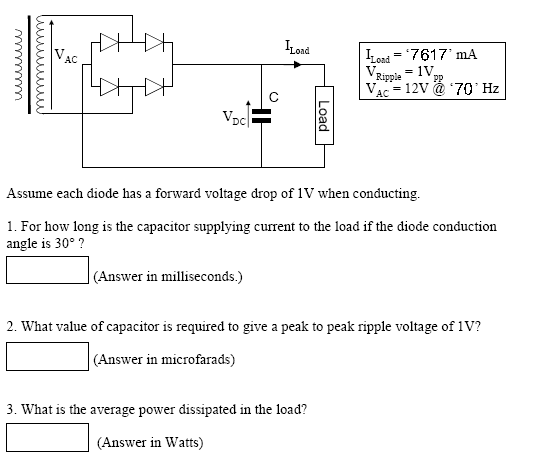# Electronics Question (University level)

## Homework StatementI'm unsure where to even start with this question so any help would be appreciated, thanks.

## Answers and Replies

I'll get you started with calculating A. After that, B and C are derived from the results found while calculating A.

We are given:
$$V_{AC}=12V_{RMS}@70Hz$$

$$I_{RMS}=7.617$$

The 'hard part' is determining voltage and frequency after full-wave rectification, 1V drop for each side of the bridge. At 30deg, only one diode drop will be applied, as it is in a half phase.

$$V_{DC}=12V_{RMS}-D_{1,2}-D_{3,4}=10V$$ *IF CORRECT - Use For (B) and (C) *

To find how long the capacitor must supply voltage, we need to find the voltage at 30 deg.
$$V=V_{PEAK}(sin\Theta)=(12*\sqrt{2})(\sin(30)=8.485V-1V=7.485$$

Now we need to calculate how many degrees there are until the voltage = 10V.
$$sin(\Theta)=\frac{V}{V_{PEAK}}=\frac{7.485}{10*1.414}=0.707$$

$$asin(0.707) = 45^\circ$$

Frequency is now 140Hz due to full wave rectification, so it has a period of about 7.143mS.
You need to calculate the time it takes for 15 degrees of voltage. You might have the Ripple, Current, and voltage to calculate B and C now*. I wasn't going to do it all for you.

Hopefully, the information above rings some bells and you can check your book to verify the formulas, and to find formulas for B and C.

*There may be an error above just to make sure you aren't copying a free answer. Quote this post to see how to write math on the forum, the sigma character to the right of the advanced reply box gives you a menu.

Ok well I asked this question on another forum and this is the reply I got, my answer follows this.

Perhaps ask yourself some questions such as :-

Can I explain how this circuit works? What is the function of each component? What is happening at different stages of the supply AC cycle?

If the frequency is 70 Hz what is the period (in ms) of the AC cycle?

If the AC cycle is also equivalent to 360° how many ms are equivalent to 30°?

If the diode conducts for 30° of the AC cycle then for how many degrees does the capacitor supply current to the load?

If I know the no. of degrees during which the capacitor supplies load current what is that equivalent to in ms?

Ok so 1/f is the period which is 14.29ms. Then 30 is 8.333% of 360 so 8.333% of 14.29ms is 1.19ms and thats how long it conducts for right? So it supplies current to the load for 13.1ms?

For the second part I tried to put something together with the equations I could find in my notes >

Vpp = Discharge Time x (dVload/dt)

and (dVload/dt) = Iload/C

So C = Iload/(Vpp/Discharge Time) seems right but not sure how to find the discharge time, would that just be the period?

If so, C = 108.8mF ? Don't have a clue on how to get the power at the last question.

Remember, the sinewave has been fullwave rectified, so the frequency has effectly doubled.

You are on the right track with the ripple voltage.

Power = V * A, or I²R, using the 10V and current given.

I'd like to see you understand the process, rather than patch together advice from different people to get by. I'm not trying to be mean.

If anything I posted doesn't make sense, please ask!